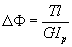TORSION

Basic kinematics of torsion: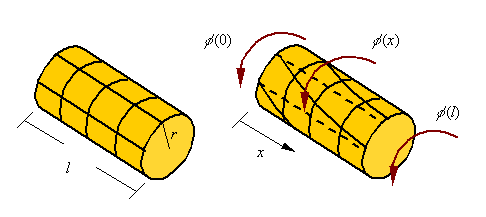It is assumed that when twisted each cross-section in a circular bar rotates as a rigid body. If the amount of rotation of each cross-section is given by the function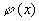, the total twistin the bar of length l shown is given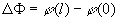During the torsion of a bar, axial lines drawn on the surface of a bar become helical and circumferential lines remain circumferential. Therefore, as can be seen in the figure, material elements are sheared in the process. This shear strain must be accompanied by a shear stress (from Hook’s law we know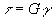).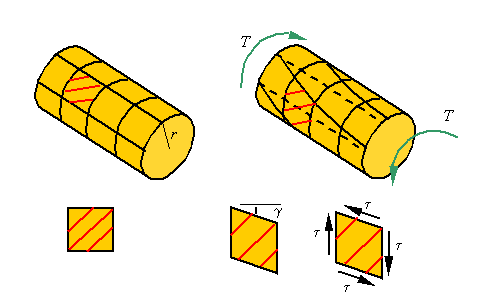The shear strain can be related to the rotation of the cross sections. If over an increment of length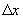, the cross section rotates an amount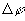, then as can be seen in the figure the shear strain is given by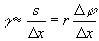Therefore, at the limit one can write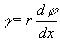As a result, the shear strain starts at zero at the center and reaches a maximum at the outer radius.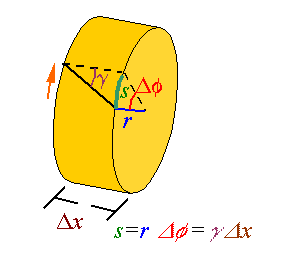The shear stress:

The shear stress, through Hook’s law, is related to the shear strain. Therefore, the shear stress is given by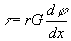Again, like the shear strain, the shear stress is zero at the core of the bar and increases linearly to a maximum at the outer surface.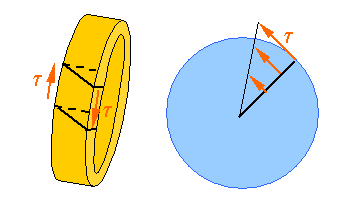Calculating the torque from the shear stress:

The torque transmitted through the cross-section of a bar is the resultant of the moments created by the shear stresses on the cross-section.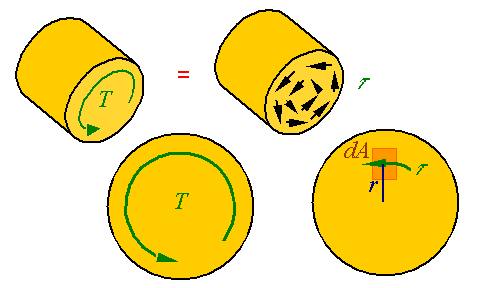The differential shear load dV that results from the shear stress applied over the area dA of the cross section is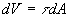. The moment created by such a shear load is dM = rdV. The equivalent torque T on the cross section is the resultant of all the moments crated by the shear stresses so that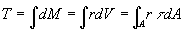Using the expression for shear stress in terms of twist in the bar we get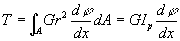where Ip is the polar area moment of inertia of the cross section. Using this relation, the expression for shear stress in terms of the torque follows fromTotal twist in a bar:

The total twist in a bar is given by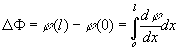The twist per unit length in terms of the torque is given by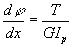Therefore, the total twist can be calculated from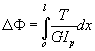In the case of a uniform bar for which the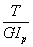is constant one can move the argument out and integrate to get